Indefinite and Definite Integrals

We want to find the indefinite integral of one of the usual textbook examples, e.g.:

> f := x^2*sin(x)*exp(-3*x);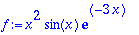> int(f, x);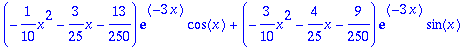To test the result, we differentiate it:

> diff(%,x);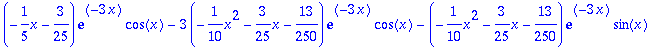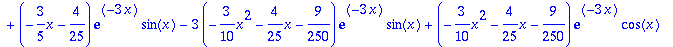> simplify(%);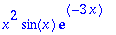Next we try a rational function:

> g := (2*x^2-3*x+1)/(x^5-7*x^4+17*x^3-19*x^2+16*x-12);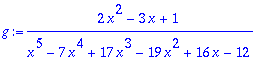> int(g, x);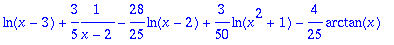To see what Maple did, we look at the partial fraction decomposition:

> factor(g);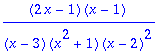> convert(g, parfrac, x);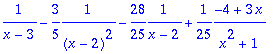Finally we want to compute the circumference of an ellipse of excentricity eps:

The parameter form of the ellipse leads to the following integral for the circumference:

> f1 := sqrt(1+eps^2*sin(t)^2);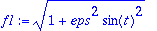> l_ell := 4*int(f1, t=0..Pi/2);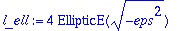The integral can not be solved with the usual functions. Since it appears in many different contexts, one has given a special name to it: "complete elliptic integral of the second kind". We look at its taylor expansion and function graph:

> taylor(%, eps=0, 5);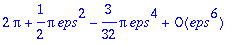For the plot of the approximation we have to throw away the O(..) term:

> l_approx := convert(%, polynom);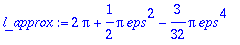> plot({l_ell,l_approx}, eps=-2..2);